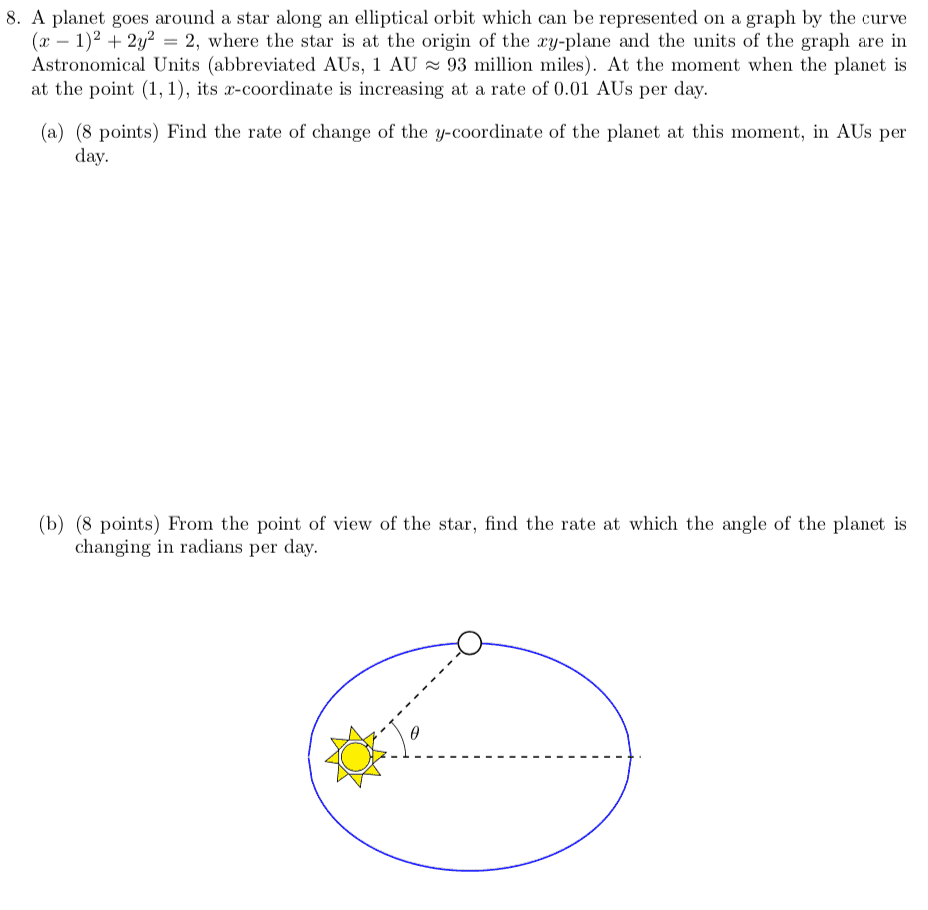# How would you solve this Related Rates problem?

• MHB
• zimsam

#### zimsam(a) $\dfrac{d}{dt}\bigg[(x-1)^2+ 2y^2=2 \bigg]$

you are given $\dfrac{dx}{dt}$ and the position of the planet.

use the derivative to calculate $\dfrac{dy}{dt}$

(b) hint …

$\theta = \arctan\left(\dfrac{y}{x}\right)$

(a) $\dfrac{d}{dt}\bigg[(x-1)^2+ 2y^2=2 \bigg]$

you are given $\dfrac{dx}{dt}$ and the position of the planet.

use the derivative to calculate $\dfrac{dy}{dt}$

(b) hint …

$\theta = \arctan\left(\dfrac{y}{x}\right)$

When I find dy/dt, it comes out to =0 for me. dy/dx=(-dx/dt(x-1))/2y. Surely the rate of change of y is not zero...

is that so … ?

View attachment 11130

I already know that dy/dt must be changing as well...
How did I make a mistake in my implicit differentiation?

I already know that dy/dt must be changing as well...
How did I make a mistake in my implicit differentiation?

you didn’t make a mistake …

dy/dt = 0 at (1,1) which is at the endpoint of the minor axis

y is changing from increasing to decreasing w/respect to time at that position